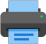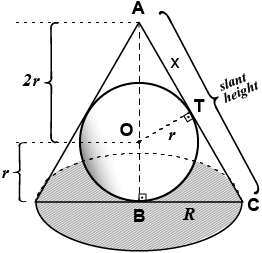Lista de exercícios do ensino médio para impressãoSelect all.
print selected exercises
Find the lateral area, the total area and the volume of a circular cone that is circumscribed to a sphere of radius $\,r\,$ and whose axial cut is an equilateral triangle.answer: $\,S_{\text lat}\,=\,6\,\pi\,r^2\,$; $\,S_{\text total}\,=\,9\,\pi\,r^2\,$; $\,V_{\text olume}\,=\,3\,\pi\,r^3\,$
×